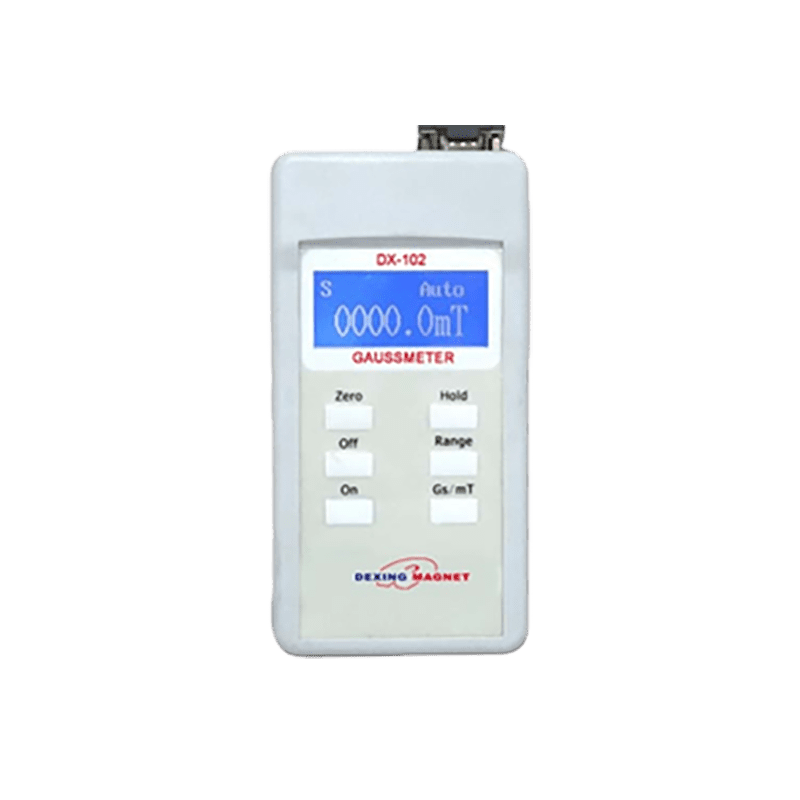#### products categories

• Tel : 18030236818
• Fax : +86-592 5237901
• Email : dexing@china-dexing.com

# The principle and function of Gauss Meter

• Gaussimeter (Tesla meter) has a wide range of applications in measuring magnetic field. This instrument is composed of Hall probe as sensor and instrument machine. The size, performance and package structure of the hall element in the probe play a key role in the accuracy of magnetic field measurement.

•• ## What is Gaussmeter ?

Gauss meter, is to measure the static or dynamic (AC) magnetic induction intensity of an object at a point in space, by Hall sensor (higher accuracy can choose fluxgate sensor). After the object magnetic field line through the generation of current voltage, the main equipment above the display magnetic induction intensity. Gaussimeter (also known as Tesla meter) is an instrument for measuring magnetic induction based on Hall effect principle. It is composed of Hall probe and measuring instrument.

## What does a Gaussmeter detect?

Gauss meter is a special instrument for detecting magnetic induction intensity of magnets. It is one of the most widely used measuring instruments in the field of magnetic measurement. It is used to measure the static or dynamic (AC) magnetic induction intensity of an object at a point in space, which is determined by Hall sensor (fluxgate sensor can be selected for higher accuracy). After the object magnetic field line through the generation of current voltage, the main equipment above the display magnetic induction intensity. Capable of measuring constant magnetic fields, DC and AC magnetic fields and pulsed magnetic fields.

## Which effect is used in Gaussmeter instrument?

Gaussimeter is an instrument that uses Hall effect to measure magnetic induction intensity B. It is confirmed by the selected Hall elements, i.e. the KP H, and the control current J is kept constant, so the Hall voltage ‰ is proportional to the measured magnetic induction intensity B. If the Gaussian scale is calibrated on the instrument panel in advance according to the size of Hall voltage, the value of magnetic induction intensity B can be directly read out from the value of the pointer when in use.

## What is gauss meter unit?

Gauss Gauss, short for height, is a non-international unit of magnetic induction. Named in honor of the German physicist and mathematician Gauss. If a length of wire is placed in a magnetic field with uniform magnetic induction intensity, and the direction is perpendicular to the direction of magnetic induction intensity, when a steady current of 1 electromagnetic system unit is passed through the long straight wire, the electromagnetic force is 1 dyne per centimeter length of the wire, then the magnetic induction intensity is defined as 1 Gaussian. Gauss is a magnetic field unit, but it's not a standard international unit, which is a Tesla.

## How does the Gaussmeter work?

Gauss sensor is the most critical component of Gauss meter, which is essentially a Hall probe. It can be planar to measure the transverse magnetic field, or axial to measure an area parallel to the probe, such as that found inside a solenoid. Probes are usually secured with brass to protect them from extreme conditions, mainly when developed for measuring small areas.

The meter transmits a test current through the probe, causing the Hall effect to generate a voltage, which the meter records. Since magnetic fields are rarely static and voltages vary, meters often have the ability to freeze readings at specific values, capture and save readings, and record only the highest voltage. Some meters can automatically resolve the difference between AC/DC fields and determine the root mean square (RMS) of the AC field.

## What are the main functions of the Gaussimeter?

What are the main functions of the Gaussimeter?

Automatic zeroing: Reset the current reading even if there is a magnetic field.

Hold function: Freezes the current value on the screen.

Peak retention: This feature displays only the highest readings collected by the Gauss meter during use.

Data capture: Allows you to save values from past records.

DC: reads the DC magnetic field current.

DC Peak (Max) : Records the maximum positive Peak reading of the DC field.

AC RMS: Collects the middle square of the entry root.

Peak AC RMS (Max) : indicates the maximum positive value collected.

The Gauss meter can read two units of measurement: Gauss and Tesla.